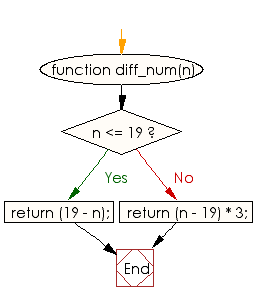# JavaScript: Compute the absolute difference between a specified number and 19

## JavaScript Basic: Exercise-17 with Solution

Write a JavaScript program to compute the absolute difference between a specified number and 19. Returns triple their absolute difference if the specified number is greater than 19.

Sample Solution:

HTML Code:

``````<!DOCTYPE html>
<html>
<meta charset="utf-8">
<meta name="viewport" content="width=device-width">
<title>JavaScript program to compute the absolute difference between a specified number and 19.  Returns triple their absolute difference if the specified number is greater than 19.</title>
<body>

</body>
</html>
```
```

JavaScript Code:

``````function diff_num(n) {
if (n <= 19) {
return (19 - n);
}
else
{
return (n - 19) * 3;
}
}

console.log(diff_num(12));
console.log(diff_num(19));
console.log(diff_num(22));
```
```

Sample Output:

```7
0
9
```

Flowchart:ES6 Version:

``````function diff_num(n) {
if (n <= 19) {
return (19 - n);
}
else
{
return (n - 19) * 3;
}
}

console.log(diff_num(12));
console.log(diff_num(19));
console.log(diff_num(22));
``````

Live Demo:

See the Pen JavaScript: Absolute difference- basic-ex-17 by w3resource (@w3resource) on CodePen.

Improve this sample solution and post your code through Disqus

What is the difficulty level of this exercise?

Test your Programming skills with w3resource's quiz.

﻿

## JavaScript: Tips of the Day

Checks if a string is an anagram of another string (case-insensitive, ignores spaces, punctuation and special characters)

Example:

```const isAnagram = (str1, str2) => {
const normalize = str =>
str
.toLowerCase()
.replace(/[^a-z0-9]/gi, '')
.split('')
.sort()
.join('');
return normalize(str1) === normalize(str2);
};
console.log(isAnagram('iceman', 'cinema')); // true
```

Output:

```true
```